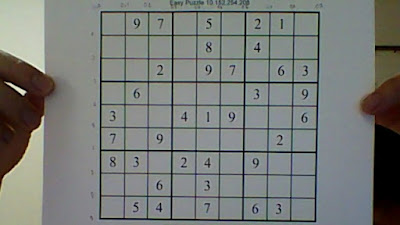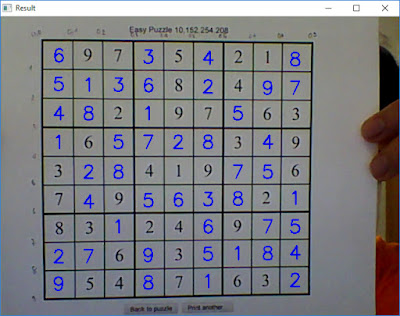# Building a simple SUDOKU Solver from scratch - Part 3: Putting in together

In part 2 of this series (See part 2), we’ve discussed how to recognize number from each cell in SUDOKU grid. With the help of SVM, we can easily recognize the digit number from SUDOKU image. Base on that recognition result, the SUDOKU grid can be solved by using the backtracking method.

First, let’s reform the recognition result to the matrix for the easier intuitive. The recognition result of a SUDOKU picture will be formed as a matrix like an example below.[0 9 7 0 5 0 2 1 0]
[0 0 0 0 8 0 1 0 0]
[0 0 2 0 9 7 0 6 3]
[0 6 0 0 0 0 3 0 9]
[3 0 0 4 1 9 0 0 6]
[7 0 9 0 0 0 0 2 0]
[8 3 0 2 4 0 9 0 0]
[0 0 6 0 3 0 0 0 0]
[0 5 4 0 7 0 6 3 0]
We assume the matrix of result after recognition (0 means the cell is empty)

We now have the “raw” recognition result as a matrix. The easiest solution to solve SUDOKU matrix is using the Backtracking algorithm. The SUDOKU can be solved by assigning every number to an empty cell. We check whether if it is safe to assign that number. After checking, we recursively check whether this assignment leads to a solution or not. If the assignment doesn’t lead to a solution, then we try the next number for current empty cell.

This post used the idea from http://www.geeksforgeeks.org/backtracking-set-7-suduku/ to solve the SUDOKU problem.
Here is the code for solving SUDOKU problem

import numpy as np
#Finding unsigned cell
#File name: SUDOKU.py
def FindUnsignedLocation(Board, l):
for row in range(0,9):
for col in range(0,9):
if (Board[row][col] == 0):
l = row
l = col
return True
return False

# Check the safety of each box in a row
def InRow(Board, row, num):
for i in range(0,9):
if (Board[row][i] == num):
return True
return False

# Check the safety of each box in a column
def InCol(Board, col, num):
for i in range(0,9):
if (Board[i][col] == num):
return True
return False

# Check the safety of a 3x3 box
def InBox(Board, row, col, num):
for i in range(0,3):
for j in range(0,3):
if (Board[i + row][j + col] == num):
return True
return False

# Check the safety of a position
def isSafe(Board, row, col, num):
return not InCol(Board, col, num) and not InRow(Board, row, num) and not InBox(Board, row - row % 3, col - col % 3, num)

def SolveSudoku(Board):
l=[0,0]
if (not FindUnsignedLocation(Board, l)):
return True
row = l
col = l
for num in range(1,10):
if (isSafe(Board, row, col, num)):
Board[row][col] = num
if (SolveSudoku(Board)):
print Board
break
Board[row][col] = 0
return False

Board = [[0, 9, 7, 0, 5, 0, 2, 1, 0],
[0, 0, 0, 0, 8, 0, 4, 0, 0],
[0, 0, 2, 0, 9, 7, 0, 6, 3],
[0, 6, 0, 0, 0, 0, 3, 0, 9],
[3, 0, 0, 4, 1, 9, 0, 0, 6],
[7, 0, 9, 0, 0, 0, 0, 2, 0],
[8, 3, 0, 2, 4, 0, 9, 0, 0],
[0, 0, 6, 0, 3, 0, 0, 0, 0],
[0, 5, 4, 0, 7, 0, 6, 3, 0]]

SolveSudoku(Board)


We use the $$9x9$$ matrix as an input of the above code. You can test with your own matrix with the above code to see the code runs properly.

Combining with the code from Part 2, we can now solve the SUDOKU and show the result like this.Fig. 1. Result of the final SUDOKU Solver

Here is the final code

## Simple SUDOKU Solver for OpenCV 2.4.13
## Visit this link for the version with OpenCV 3.x: https://github.com/huuquan1994/Sudoku-Solver/blob/master/Main_SUDOKU_OpenCV3.py

import cv2
import numpy as np
import joblib
import SUDOKU

font = cv2.FONT_HERSHEY_SIMPLEX
ratio2 = 3
kernel_size = 3
lowThreshold = 30
count = 1
# Load the pre-trained SVM model.
# Please note that you will need to train a new classifier if you run this code under Python 3.x

is_print = True

cv2.namedWindow("SUDOKU Solver")
vc = cv2.VideoCapture(0)
if vc.isOpened(): # try to get the first frame
else:
rval = False
while rval:
sudoku1 = cv2.cvtColor(frame, cv2.COLOR_BGR2GRAY)
sudoku1 = cv2.blur(sudoku1, (1,1))
edges = cv2.Canny(sudoku1, lowThreshold, lowThreshold*ratio2, kernel_size)
lines = cv2.HoughLines(edges, 2, cv2.cv.CV_PI /180, 300, 0, 0)
if (lines is not None):
lines = lines
lines = sorted(lines, key=lambda line:line)
diff_ngang = 0
diff_doc = 0
lines_1=[]
Points=[]
for rho,theta in lines:
a = np.cos(theta)
b = np.sin(theta)
x0 = a*rho
y0 = b*rho
x1 = int(x0 + 1000*(-b))
y1 = int(y0 + 1000*(a))
x2 = int(x0 - 1000*(-b))
y2 = int(y0 - 1000*(a))
if (b>0.5):
if(rho-diff_ngang>10):
diff_ngang=rho
lines_1.append([rho,theta, 0])
else:
if(rho-diff_doc>10):
diff_doc=rho
lines_1.append([rho,theta, 1])
for i in range(len(lines_1)):
if(lines_1[i] == 0):
for j in range(len(lines_1)):
if (lines_1[j]==1):
theta1=lines_1[i]
theta2=lines_1[j]
p1=lines_1[i]
p2=lines_1[j]
xy = np.array([[np.cos(theta1), np.sin(theta1)], [np.cos(theta2), np.sin(theta2)]])
p = np.array([p1,p2])
res = np.linalg.solve(xy, p)
Points.append(res)

if(len(Points)==100):
result = []
board = []
for i in range(0,9):
for j in range(0,9):
y1=int(Points[j+i*10]+5)
y2=int(Points[j+i*10+11]-5)
x1=int(Points[j+i*10]+5)
x2=int(Points[j+i*10+11]-5)
X = sudoku1[y1:y2,x1:x2]
if(X.size!=0):
X = cv2.resize(X, (36,36))
num = clf.predict(np.reshape(X, (1,-1)))

#Collect the result
result.append(num)
board.append(num)

# Reshape to 9x9 matrix
result = np.reshape(result, (9,9))
board = np.reshape(board, (9,9))

# Solve the SUDOKU grid
if(SUDOKU.SolveSudoku(result)):
# If it can solve SUDOKU matrix, show the result
for i in range(0,9):
for j in range(0,9):
if(array[i][j]==0):
cv2.putText(frame,str(result[i][j]),(int(Points[j+i*10+10]+15),
int(Points[j+i*10+10]-10)),font,1,(225,0,0),2)
# Show the result picture
cv2.imshow("Result", frame)

cv2.imshow("SUDOKU Solver", frame)
key = cv2.waitKey(20)
if key == 27: # exit on ESC
break
vc.release()
cv2.destroyAllWindows()


Here is the end of this SUDOKU Solver series. It’s just the simple SUDOKU solver from taken images. I hope that you can improve this code or think about the new ideas.

If you have any questions or comments, please feel free to contact me by email. All the training data and source code can be found in this GitHub link: https://github.com/huuquan1994/Sudoku-Solver